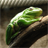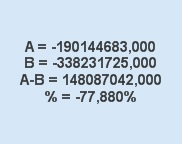# QlikView App Dev

Discussion Board for collaboration related to QlikView App Development.

Announcements
LINKEDIN LIVE: Democratizing data to enhance customer-centricity. JULY 29TH REGISTER TODAY
cancel
Showing results for
Did you mean:Master III

## Get values in percentage

Hi,

i used below code to get values in percentage

,'#,##0%')

but this what i get

106,080,500,56%

what i am doing wrong here i used num function

and Total also

plz suggest something

thanks

25 RepliesCreator II

How much is A approximately?

and how much is B approximately?Master III
Author

A=-190,144,683

B=-338,231,725MVP

Like Linus suggests, put the parts of the big expression as separate expressions in a text box. For example:

='A = ' & (ExpressionA) & chr(10) &

'B = ' & (ExpressionB)

Also note that when applying num() with a format string that includes a %-symbol requires an expression result between 0 and 1 to get 0-100%. The expression should be (A-B)/A instead of (A-B)/A*100.

Also check the format string for correct decimal and thousand separators. It looks like QV is messing up the output due to separator misinterpretations.

Best,

PeterCreator II

Ok good. The negative values make it a little bit more difficult to follow along but what we see is that

-190/-338 = 0.56. A is 56 percent of B.

the difference of B-A = -148 in percent /-338=0.44Master III
Author

like this peter

=num(sum({<ACNT_FLEX_02={'Balance Sheet'}>}TBAL_MTD_DR_LC_1-TBAL_MTD_CR_LC_1) & Chr(10) -

num(sum({<ACNT_FLEX_02={'Balance Sheet'}>}TBAL_MTD_DR_LC_1-TBAL_MTD_CR_LC_1)*100,'#,##0%'))

it gives me blank valueMaster III
Author

can u tell me so how do it?

thanksMVP

Hmmm, maybe this sheds some light from another direction:

This text box expression:

='A = ' & num(-190144683, '#,##0') & chr(10) &

'B = ' & num(-338231725, '#,##0') & chr(10) &

'A-B = ' & num(-190144683+338231725, '#,##0') & chr(10) &

'% = ' & num((-190144683+338231725)/-190144683, '#,##0%')

with default separators

SET ThousandSep='.';

SET DecimalSep=',';

produces this resultMVP

Sorry, that's no what I meant. See below for an example.

BTW if you get a blank, then there is something wrong with the expression itself and not necessarily with the formatting.Master III
Author

thanks i will check and let u knowMaster III
Author

i did this

=num((sum({<ACNT_FLEX_02={'Balance Sheet'}>}TBAL_MTD_DR_LC_1-TBAL_MTD_CR_LC_1) -